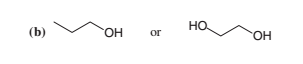# Problem: Which compound in each of the following pairs would have the higher boiling point? Explain your answers.

###### FREE Expert Solution
90% (487 ratings)
###### Problem Details

Which compound in each of the following pairs would have the higher boiling point? Explain your answers.Frequently Asked Questions

What scientific concept do you need to know in order to solve this problem?

Our tutors have indicated that to solve this problem you will need to apply the Physical Properties of Alcohols concept. If you need more Physical Properties of Alcohols practice, you can also practice Physical Properties of Alcohols practice problems.

What is the difficulty of this problem?

Our tutors rated the difficulty ofWhich compound in each of the following pairs would have the...as low difficulty.

What professor is this problem relevant for?

Based on our data, we think this problem is relevant for Professor Saslow's class at TAMU.

What textbook is this problem found in?

Our data indicates that this problem or a close variation was asked in Organic Chemistry - Solomons 10th Edition. You can also practice Organic Chemistry - Solomons 10th Edition practice problems.